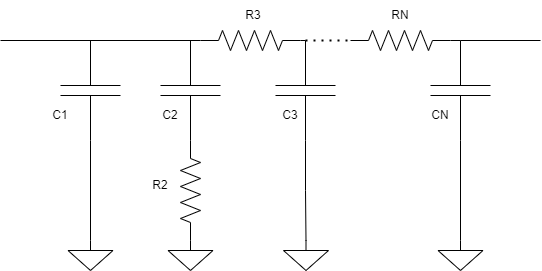# Loop Filter

Model second-, third-, or fourth-order passive loop filter

Since R2019a

•Libraries:
Mixed-Signal Blockset / PLL / Building Blocks

## Description

The Loop Filter subsystem block is a passive filter whose order can vary from two to four. In a phase-locked loop (PLL) system, the subsystem filters the output of Charge Pump block and delivers the control voltage to a VCO block to generate required frequency signal.

nth Order Passive Loop Filter## Ports

### Input

expand all

Input current, specified as a scalar. In a phase-locked loop (PLL) system, the in port is connected to the output of a Charge Pump block, which provides the current value.

Data Types: `double`

### Output

expand all

Output voltage, specified as a scalar. In a PLL system, the out port is connected to the input port of a VCO block and provides the control voltage to VCO.

Data Types: `double`

## Parameters

expand all

### Configuration

Order of the loop filter. Simulates a second-, third-, or fourth-order passive RC loop filter.

#### Programmatic Use

• Use `get_param(gcb,'FilterType')` to view the current order of Loop filter type.

• Use `set_param(gcb,'FilterType',value)` to set Loop filter type to a specific order.

Capacitor value C1, specified as a positive real scalar in farads.

#### Programmatic Use

• Use `get_param(gcb,'C1')` to view the current value of C1 (F).

• Use `set_param(gcb,'C1',value)` to set C1 (F) to a specific value.

Data Types: `double`

Capacitor value C2, specified as a positive real scalar in farads.

#### Programmatic Use

• Use `get_param(gcb,'C2')` to view the current value of C2 (F).

• Use `set_param(gcb,'C2',value)` to set C2 (F) to a specific value.

Data Types: `double`

Capacitor value C3, specified as a positive real scalar in farads.

#### Dependencies

To enable this parameter, select ```3rd Order passive``` or ```4th Order passive``` in Loop filter type.

#### Programmatic Use

• Use `get_param(gcb,'C3')` to view the current value of C3 (F).

• Use `set_param(gcb,'C3',value)` to set C3 (F) to a specific value.

Data Types: `double`

Capacitor value C4, specified as a positive real scalar in farads.

#### Dependencies

To enable this parameter, select ```4th Order passive``` in Loop filter type.

#### Programmatic Use

• Use `get_param(gcb,'C4')` to view the current value of C4 (F).

• Use `set_param(gcb,'C4',value)` to set C4 (F) to a specific value.

Data Types: `double`

Resistor value R2, specified as a positive real scalar in ohms.

#### Programmatic Use

• Use `get_param(gcb,'R2')` to view the current value of R2 (ohms).

• Use `set_param(gcb,'R2',value)` to set R2 (ohms) to a specific value.

Data Types: `double`

Resistor value R3, specified as a positive real scalar in ohms.

#### Dependencies

To enable this parameter, select ```3rd Order passive``` or ```4th Order passive``` in Loop filter type.

#### Programmatic Use

• Use `get_param(gcb,'R3')` to view the current value of R3 (ohms).

• Use `set_param(gcb,'R3',value)` to set R3 (ohms) to a specific value.

Data Types: `double`

Resistor value R4, specified as a positive real scalar in ohms.

#### Dependencies

To enable this parameter, select ```4th Order passive``` in Loop filter type.

#### Programmatic Use

• Use `get_param(gcb,'R4')` to view the current value of R4 (ohms).

• Use `set_param(gcb,'R4',value)` to set R4 (ohms) to a specific value.

Data Types: `double`

Select to enable increased buffer size during simulation. This increases the buffer size of the Convert Sample Time subsystem inside the Loop Filter block. By default, this option is deselected.

Number of samples of the input buffering available during simulation, specified as a positive integer scalar. This sets the buffer size of the Convert Sample Time subsystem inside the Loop Filter block.

Selecting different simulation solver or sampling strategies can change the number of input samples needed to produce an accurate output sample. Set the Buffer size to a large enough value so that the input buffer contains all the input samples required.

#### Dependencies

This parameter is only available when Enable increased buffer size option is selected in the Configuration tab.

#### Programmatic Use

• Use `get_param(gcb,'NBuffer')` to view the current value of Buffer size.

• Use `set_param(gcb,'NBuffer',value)` to set Buffer size to a specific value.

Data Types: `double`

### Impairments

Select to add circuit impairments to simulation. By default, this option is disabled.

Temperature of the resistor, specified as a scalar in degree Celsius. Operating temperature (℃) determines the level of thermal (Johnson) noise.

#### Dependencies

To enable this parameter, select Enable impairments in the Impairments tab.

#### Programmatic Use

• Use `get_param(gcb,'Temperature')` to view the current value of Operating temperature (℃).

• Use `set_param(gcb,'Temperature',value)` to set Operating temperature (℃) to a specific value.

Data Types: `double`